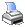﻿ MCL Reference > Arithmetic Functions > Sum( )
 Navigation:  MCL Reference > Arithmetic Functions > Sum( )The Sum( ) function returns the sum of a set of values. Null values inside the set will be ignored. You may pass up to 27 arguments into the function.

Syntax

Sum(x1,x2,x3,...)

x1, x2, x3,... must be numbers.

Returns

Numeric

Examples

Sum(Column('This Rating'),Column('Last Rating'))

The sum of the columns This Rating and Last Rating will be returned.

Sum(12, .NULL., 24)

36 will be returned by the above function. .NULL. is ignored.

Sum(12, 0, 24)

36 will be returned by the above function.